# Mensuration class 8 solutions 11.2

In this page we have mensuration class 8 solutions 11.2 . This exercise has questions about Area of trapezium, Area of general quadrilaterals and Area of polygon.Hope you like them and do not forget to like , social share and comment at the end of the page.

## Mensuration class 8 solutions 11.2

Question 1
The shape of the top surface of a table is a trapezium. Find its area if its parallel sides are 1 m and 1.2 m and perpendicular distance between them is 0.8 m.
Area of Trapezium is given by
= (1/2) × (Sum of parallel sides) × Perpendicular distance
Here in this question, Perpendicular distance=.8  m
Parallel sides are 1m and 1.2 m
So Area of Trapezium
= (1/2) × (1+1.2) ×.8
=.88 m2

Question 2
The area of a trapezium is 34 cm² and the length of one of the parallel sides is 10 cm and its height is 4 cm. Find the length of the other parallel side.
Area of Trapezium
= 1/2 x sum of parallel sides x perpendicular distance
Here the givens are
Area of a trapezium = 34 cm²
Length of one parallel side =10cm
Height=4 cm
Let a be the other parallel side, then
34= (1/2) x(10+a) x4
34=2(10+a)
10+a=17
a=17-10=7 cm

Question 3
Length of the fence of a trapezium shaped field ABCD is 120 m. If BC = 48 m, CD = 17 m and AD = 40 m, find the area of this field. Side AB is perpendicular to the parallel sides AD and BC.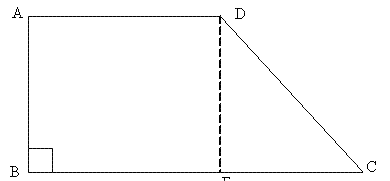Area of Trapezium
= 1/2 x sum of parallel sides x perpendicular distance
Given
Parallel sides are given but height is missing
If a perpendicular from D to BC is drawn then it will be equal to AB,
Then EC = 48-40 = 8 m.
In ΔDEC, By Pythagoras theorem
DE2 + EC2= DC2
Or
DE2 = DC2 - EC2
= 172 - 82 = 289 - 64 = 225
Or, DE = 15 m
Area of Trapezium
= (1/2) (48+40) 15
=660 m2

Question 4
The diagonal of a quadrilateral shaped field is 24 m and the perpendiculars dropped on it from the remaining opposite vertices are 8 m and 13 m. find the area of the field.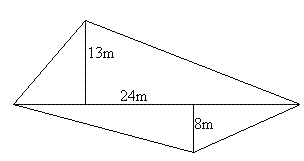= Area of Upper Triangle + Area of Upper Triangle
Area of Triangle is given by
=(1/2) ×Height ×Base
So Area of Upper Triangle
=(1/2) ×13×24= 156 m2
Area of Lower Triangle
=(1/2) ×8×24= 96 m2

So
Area of the field = 156 + 96 = 252 m2

Question 5
The diagonals of a rhombus are 7.5 cm and 12 cm. Find its area.
Area of Rhombus=  (1/2) × D1 × D2
Here
D1= 7.5 cm
D2= 12 cm
So Area of rhombus
=45 cm2

Question 6
Find the area of a rhombus whose side is 6 cm and whose altitude is 4 cm. If one of its diagonals is 8 cm long, find the length of the other diagonal.
Answer: A rhombus is special case of parallelogram
So Area of rhombus= Altitude X side= 24 cm2
Let d be the other diagonal, then
Area of Rhombus=  (1/2) × D1 × D2
24=(1/2) ×8 × a
Or a =6 cm

Question 7
The floor of a building consists of 3000 tiles which are rhombus shaped and each of its diagonals are 45 cm and 30 cm in length. Find the total cost of polishing the floor, if the cost per m² is Rs 4
Area of Rhombus=  (1/2) × D1 × D2
= (1/2) × 45 × 30=675
Now cost of Polishing the floor
= Total Area × rate
= 3000 × 675 ×4/10000
=Rs 810

Question 8
Mohan wants to buy a trapezium shaped field. Its side along the river is parallel to and twice the side along the road. If the area of this field is 10500 m2 and the perpendicular distance between the two parallel sides is 100 m, find the length of the side along the river.
Let a be the length of along the road, the length of the side along river will be 2a
Area of Trapezium is given by
= (1/2) × (Sum of parallel sides) × Perpendicular distance
= (1/2) ×(a+2a) ×100= 150a
Now Area of Trapezium= 10500 m2
10500= 150a
a=70
So sides are 70 and 140 m

Question 9
Top surface of a raised platform is in the shape of a regular octagon as shown in the figure. Find the area of the octagonal surface.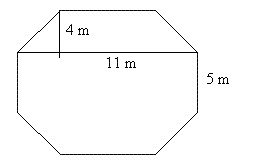Upper part is in the shape of a trapezium. This is mirrored in the lower part as well. So area of two trapeziums added to the area of middle rectangular portion will be equal to the area of the octagon.
Area of Trapezium
= (1/2) × (Sum of parallel sides) × Perpendicular distance
= (1/2) (11+5)4= 32m2

So, Area of 2 trapeziums = 64 m2
Now, Area of Rectangle = Length x Breadth
= 11 x 5 = 55
Hence, Area of the platform = 64+55 = 119 m2

Question 10
There is a pentagonal shaped park as shown in the figure. For finding its area Jyoti and Kavita divided it in two different ways. Find the area of this park using both ways. Can you suggest some other way of finding its area?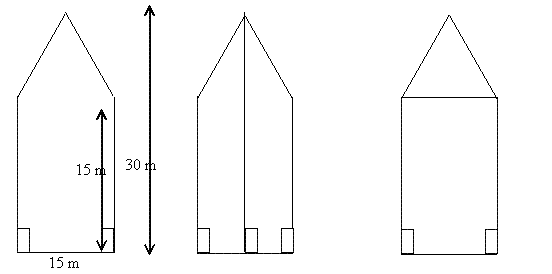Jyoti has divided the park in to congruent trapeziums. The parallel sides of the trapezium are measuring 15 m and 30 m and the perpendicular distance is 7.5 m
Area of Trapezium=
= (1/2) × (Sum of parallel sides) × Perpendicular distance
= (1/2)(30+15) 7.5= 337 m2
Kavita divided the park into upper triangular portion and lower rectangular portion. Sides of rectangle are 15 m each. Base and height of triangle are 15 m each.
Area of square= 15×15=225 m2
Area of Triangle= (1/2) ×15×15= 112.5
Total Area = 225 + 112.5 = 337.5  m2

Question 11
Diagram of the adjacent picture frame has outer dimensions =24 cm × 28 cm and inner dimensions 16 cm × 20 cm. Find the area of each section of the frame, if the width of each section is same.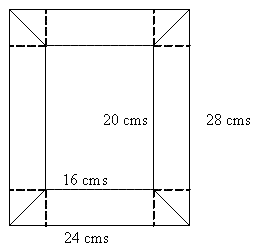Answer: Let us draw a perpendicular from any side of the inner rectangle to the side of the outer rectangle as shown above. This will give us 4 congruent triangles as 24-16 = 8 and 28-20 = 8 and width of each section is same
So Height and Base of each triangle are 4 cm each
Sides of Both Horizontal Rectangles= 4 cm and 16 cm
Sides of Both vertical Rectangles= 4 cm and 20 cm
Now of the each Triangle= (1/2) ×4×4= 8 cm2

Area of the horizontal rectangle = 4 x 16 = 64
Hence, Area of lower horizontal section of the frame = 64 + 8 + 8 = 80 cm2
Area of the vertical rectangle = 4 x 20 = 80
Hence, Area of One Vertical Section of the frame = 80 + 8 + 8 = 96  cm2

## Summary

1. Mensuration class 8 solutions 11.2 has been prepared by Expert with utmost care. If you find any mistake.Please do provide feedback on mail. You can download the solutions as PDF in the below Link also
2. This chapter 11 has total 4 Exercise 11.1 ,11.2 ,11.3 and 11.4. This is the Second exercise in the chapter.You can explore previous exercise of this chapter by clicking the link below